rexresearch.com

Fran De AQUINO
Gravitational Spacecraft

Fran de Aquino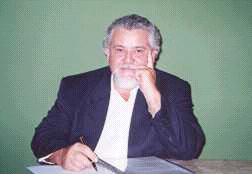Professor Fran De Aquino's Webpage -- http://www.frandeaquino.org/
http://users.elo.com.br/~deaquino/

Physics Department, Maranhao State University
deaquino@uema.br

"Controlling the Gravitational Mass of Metallic Lamina, & Gravity Acceleration above it"
( PDF ) Al foil & ELF reduces G. Note the similarity to Hooper.

http://xxx.lanl.gov/abs/gr-qc/9910036v1
http://xxx.lanl.gov/find/gr-qc/1/au:+Aquino_F/0/1/0/all/0/1

Gravitation and Electromagnetism : Correlation and Grand Unification
by
Fran De Aquino
(Maranhao State University, Brazil)

It is demonstrated that gravitational and inertial masses are correlated by an electromagnetic factor. From the practical point of view this is very important because it means the possibility of electromagnetic control of the gravity. Some theoretical consequences of the correlation are:

incorporation of Mach's principle into Gravitation Theory;
new relativistic expression for the mass ;
the generalization of Newton's second law for the motion;
the deduction of the differential equation for entropy directly from the Gravitation Theory.

Another fundamental consequence of the mentioned correlation is that, in specific ultra-high energy conditions, the  gravitational and electromagnetic fields can be described by the same Hamiltonian, i.e., in these circumstances, they are unified ! Such conditions can have occurred inclusive in the Initial Universe, before the first spontaneous breaking of symmetry.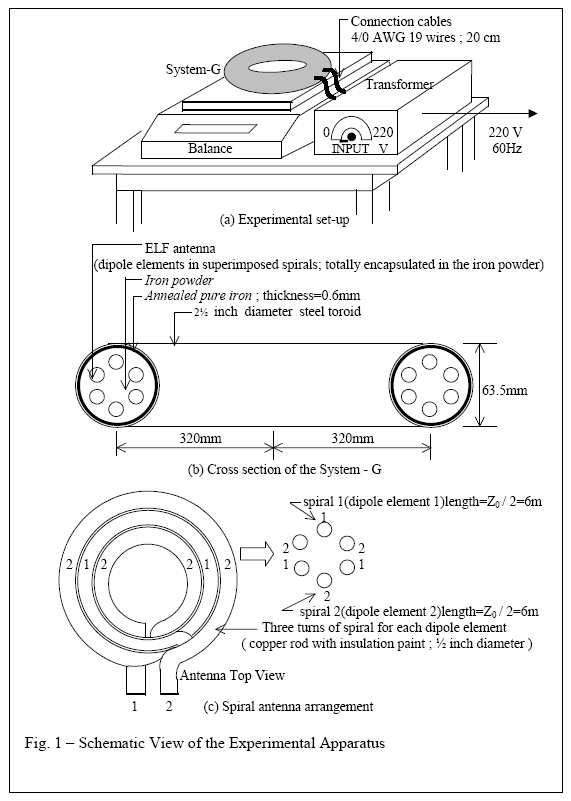JLN Labs
March 21th, 2000
Email : JNaudin509@aol.com
JLN Lab's eGroup at : jlnlabs@egroups.com if you are a team member.

The Gravitational SpaceCraft from Fran De Aquino
Warping to the deep space...
by
Fran De Aquino

It is known that photons have null inertial mass (mi = 0 ) and that they do not absorb others photons (U = 0 ). So , if we put mi = 0 and U = 0 in Eq.(1.04), the result is mg = 0. Therefore photons have null gravitational mass. Let us consider a point source of radiation with power P , frequency f and radiation density at distance r given by D = P /4p r2

Due to the null gravitational mass of the photons, it must be possible to build a shield of photons around the source, which will impede the exchange of gravitons between the particles inside the shield and the rest of the Universe. The shield begins at distance rs from the source where the radiation density is such that there will be a photon in opposition to each incident graviton . This critical situation occurs when D = hf 2 / Sg , where Sg is the geometric cross section of the graviton. Thus rs is given by the relation, rs = (rg / f )( P/h)1/2We then see that the ELF radiation are the most appropriate to produce the shield. It can be easily shown that, if f << 1mHz , the radiation will traverse any particle . It is not difficult to see that in this case, there will be "clouds" of photons around the particles inside the shield. Due to the null gravitational mass of the photons , these "clouds" will impede the exchange of gravitons between the particle inside the "cloud" and the rest of the Universe. Thus, we can say that the gravitational mass of the particle will be null with respect to the Universe, and that the space-time inside the shield (out of the particles) becomes flat or euclidean . It is clear that the space-time which the particles occupies remains non-euclidean.

In an euclidean space-time the maximum speed of propagation of the interactions is infinitebecause , as we know, the metrics becomes from Galilei.

Therefore, the interactions are instantaneous . Thus , in this space-time the speed of photons must be infinite, simply because they are the quanta of the electromagnetic interaction. So, the speed of photons will be infinite inside the shield.

On the other hand , the new relativistic expression for mass, Eq.(2.06),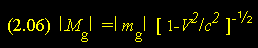shows that a particle with null gravitational mass isn’t submitted to the increase of relativistic mass, because under these circumstances its gravitational mass doesn't increase with increasing velocity .i.e., it remains null independently of the particle's velocity. In addition , the gravitational potentialor the particle will be null and, consequently , the component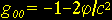of the metric tensor will be equal to -1.

Thus , we will have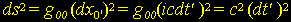where t' is the time in a clock moving with the particle , and ds2 = c2 dt2 where t is the time indicated by a clock at rest ( dx = dy = dz = 0 ).

From the combination of these two equations we conclude that t' = t .This means that the particle will be not more submitted to the relativistic effects predicted in Einstein's theory. So, it can reach and even surpass the speed of light . We can imagine a spacecraft with positive gravitational mass qual to (m) kg , and negative gravitational mass ( see System-G in appendix A) equal to - (m - 0.001) kg . It has a shield of photons , as above mentioned. If the photons, which produce the shield , radiate from the surface of the spacecraft , then the space-time that it occupies remains non-euclidean ,and consequently , for an observer in this space-time , the total gravitational mass of the spacecraft, will be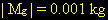. Therefore , if its propulsion system produces F=10N (only) the spacecraft acquires acceleration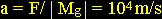( see Eq.(2.05)).Furthermore, due to the "cloud" of photons around the spacecraft its gravitational interaction with the Universe will be null , and therefore, we can say that its gravitational mass will be null with respect to the Universe. Consequently, the inertial forces upon the spacecraft will also be null, in agreement with Eq.2.05 ( Mach’s principle ).This means that the spacecraft will lose its inertial properties . In addition, the spacecraft will can reach and even surpass the speed of light because , as we have seen , a particle with null gravitational mass will be not submitted to the relativistic effects.

Fran De Aquino, April 20th, 2000

JLN Labs
The System-G from Fran De Aquino : Engineering the device...
by
Fran De Aquino and Steve Burns
March 17th, 2000

The System-G experiment has been performed successfuly by Fran De Aquino on January 27th, 2000 at the Physics Department of Maranhao State University in Brazil.

In the System-G torus, the ELF energy generated by the dipole antenna must be fully absorbed the atoms of the annealed iron lamina. The ELF energy is done by this equation below :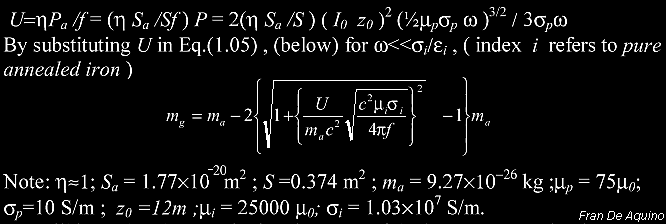The curve below, shows that a System-G weight ( 35 Kg ) can be nullified at 60Hz with a current of 400 Ampere.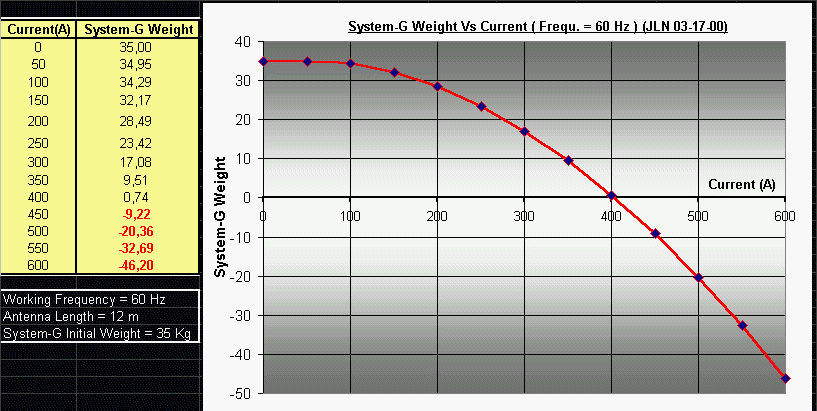The use of the power grid for the basic System-G experiment has been conducted easily by using the power grid frequency ( 60 Hz, in Brazil ), this was energy consumming ( about 11 KW ). But for improving the efficiency of the whole apparatus, Power Input Vs weight lifted, its seems much adapted to use a lower frequency than previously for the ELF signal as shown on the table below :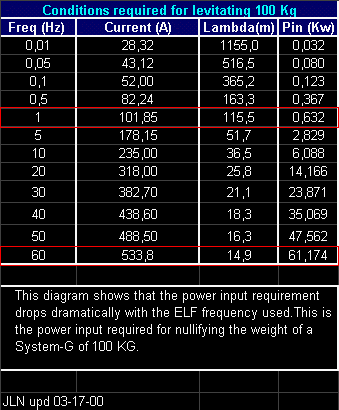You notice that, to levitate a 100 Kg weight apparatus,
at 60 Hz we need 61 KW and for the same results, only 632 W is required at 1 Hz.You may notice on the diagram above that only 1460 Watts are required to levitate 50 Kg at a frequency of 5 Hz...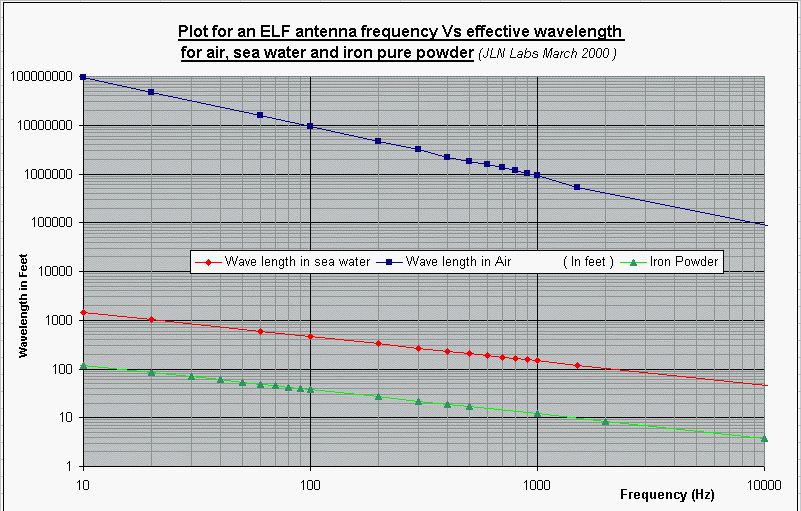The plot above, shows the phase velocity reduced wavelength for an ELF antenna
in seawater (published by the US Navy in 1960's), in air and also in a pure iron powder (for the System-G).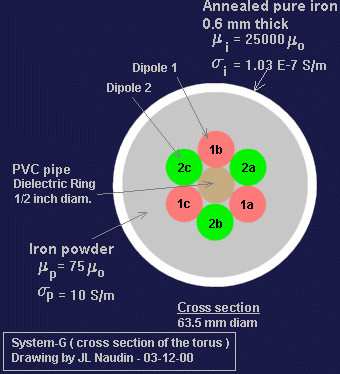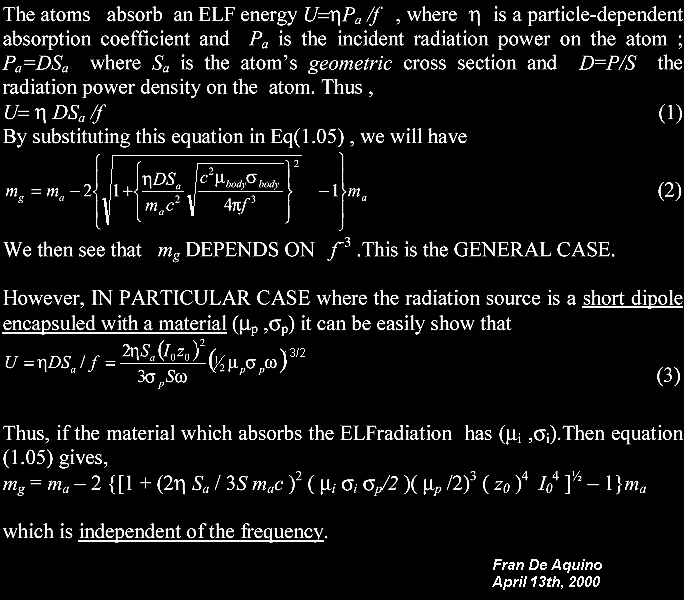JL NAUDIN LABS
03/28/00

by J-L Naudin

ZIP ]

Notes : You must have the JavaScript enabled in your Internet browser. This very helpful tool will allow you to find quickly the good setup for building your own System-G. You need only to enter the specifications of your components in the green areas and the solver will give you the calculated values in the red areas. The preset values are the parameters used by Fran De Aquino during his historical System-G experiment on January 27th, 2000, so you will able to check by yourself that the calculated values are close to the experimental values measured by Fran De Aquino.

When all your parameters are entered, you need only to press the "Calculate" button . To restart again the calculation, the "Restore" button will reset all the datas fields to their initial values.

Good Engineering,

Jean-Louis Naudin

Gravity Control by Means of Electromagnetic Field Through Gas or Plasma at Ultra-Low Pressure
by
Fran De Aquino
[ PDF ]

A Gravitational Shielding Based on ZnS:Ag Phosphor
by
Fran De Aquino
[ PDF ]

It was shown that there is a practical  possibility of gravity control on elelectroluminescent materials. We present here a type of G-SHielding based on an EL phosphor, namely zinc sulfide doped with silver ( ZnS:Ag ) which can reduce the cost of the G-Motor previously presented...How to Extract Energy Directly from a Gravitational Field
by

Fran De Aquino
[ PDF ]

Gravity is related to gravitational mass of the bodies. According to the weak form of Einstein’s General Relativity equivalence principle, the gravitational and inertial masses are equivalent. However recent calculations (gr-qc/9910036) have revealed that they are correlated by an adimensional factor, which depends on the incident radiation upon the particle. It was shown that there is a direct correlation between the radiation absorbed by the particle and its gravitational mass, independently of the inertial mass. This finding has fundamental consequences to Unified Field Theory and Quantum Cosmology. It was also shown that only in the absence of electromagnetic radiation this factor becomes equal to one and that, in specific electromagnetic conditions, it can be reduced, nullified or made negative. This means that there is the possibility of control of the gravitational mass by means of the incident radiation. This unexpected theoretical result was recently confirmed by an experiment (gr-qc/0005107). Consequently there is a strong evidence that the gravitational forces can be reduced, nullified and inverted by means of electromagnetic radiation. This means that , in practice we can produce gravitational binaries, and in this way to extract energy from a gravitational field. Here we describe a process by which energy can be extracted directly from any site of a gravitational field.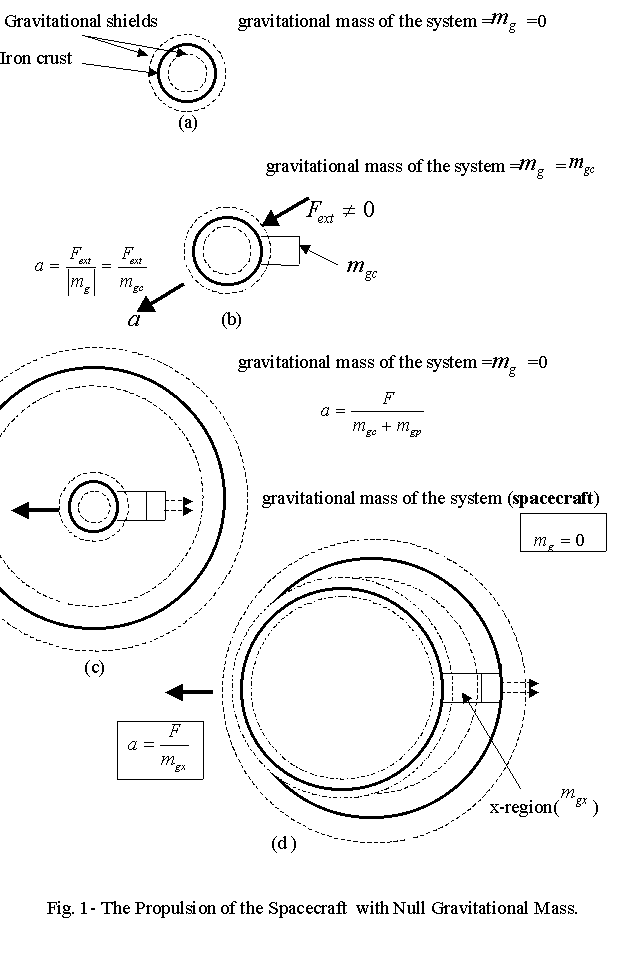http://www.frandeaquino.org/
http://users.elo.com.br/~deaquino/
Professor Fran De Aquino's Webpage

Physics Department, Maranhao State University
deaquino@uema.br

For several years, physicist Fran De Aquino worked as Senior-Researcher at the Instituto Nacional de Pesquisas Espaciais - INPE (National Institute for Space Research - INPE , Brazil) (INPE Registration Number 02844 CRN, SIAPE Registration Number 0024730). He was also Director of the INPE's Space Observatory in the city of São Luís, since the installation of this observatory until 1997.

Currently, physicist Fran De Aquino is a professor at the Universidade Estadual do Maranhão- UEMA (State University of Maranhao, Brazil), (UEMA Registration Number 5155). He also works as Consultant in Science & Technology for international companies. Fran De Aquino's publications list includes several scientific articles and books.

His research involves many relevant aspects of Gravitation and Cosmology. After thirty years of research, professor De Aquino discovered that the gravity can be quantized, starting from the generalization of the known action function. In discovering the quantization of gravity, Fran De Aquino made true an old dream of the Quantum Physicists - to obtain a Quantum Theory for Gravity.

This Quantum Theory of Gravity unifies Einstein's Theory of General Relativity with the Quantum Theory and, furthermore, it leads to an equation of correlation between gravitational mass and the inertial mass, which opens doors for gravity control technologies and the G ENERGY , the energy of the future that possibly it will change the paradigms of energy generation, transportation and telecommunications.

The theory shows that there is a revalidation of the strong equivalence principle, and consequently Einstein's equations are preserved. Thus, De Aquino's Quantum Theory of Gravity does not invalidate Einstein's Theory, on the contrary, it just complements this theory, in such a way as Einstein's theory has complemented Newton's theory.

The Einstein's equations are deduced directly from De Aquino's theory. Moreover, a generalized equation for inertial forces is obtained , which incorporates the Mach's principle into Gravitation, explainning therefore the origin of inertia, and yielding a new expression for gravitational forces which includes the Newton's attraction law, and shows that the graviton must have spin 1 and not 2. The theory also allows the deduction of the Entropy's differential equation; the Hamiltonian for a particle in an electromagnetic field and the reciprocal fine structure constant. It is also possible to explain the Inflation Period and the Hidden Matter, without assuming the existence of vacuum fluctuations. Similarly, it is possible to obtain the Casimir force, whithout assuming the existence of zero-point field. Another fundamental consequence of De Aquino's theory is the possibility of control of the gravitational mass. In addition, De Aquino's Quantum Theory of Gravity shows that, in specific ultra-high energy conditions, the gravitational and electromagnetic fields can be described by the same Hamiltonian, i.e., in these circumstances, they are unified. Such conditions might have occurred inclusively in the Initial Universe, before the first spontaneous breaking of Symmetry. Therefore, this Kinetic approach to Quantum Gravity will allow us to understand some crucial matters in Quantum Cosmology, including the early stages of the Big Bang. For some physicists, this theory might be it the Holy Graal of Modern Physics because it can complete the unification of all fundamental laws of Physics.

Now, it is possible for the first time to write down a single equation (Eq.1 of Mathematical Foundations of the Relativistic Theory of Quantum Gravity. See T-shirt below) that can explain all the laws of physics( including the Einstein's equations), all the forces of nature - the proverbial "theory of everything". This grand unified theory comprhends Philosophy, God (including feminine aspects), and the Soul. It is not restricted only to the material world it includes not only force particles and matter particles, not only general relativity and Quantum Gravity, but also a theory of consciousness.

http://users.elo.com.br/~deaquino/Kinetic.pdf
http://arxiv.org/abs/physics/0212033
http://cdsweb.cern.ch/ ( Record # 595902 )
http://issuu.com/aquino/docs/kinetics
http://www.scribd.com/doc/21561154/Mathematical-Foundations-of-the-Relativistic-Theory-of-Quantum-Gravity
http://sciencestage.com/d/5856128/mathematical-foundations-of-the-relativistic-theory-of-quantum-gravity.html

Mathematical Foundations of the Relativistic Theory of Quantum Gravity
by
Fran De Aquino

[ PDF ]

Abstract: Starting from the action function, we have derived a theoretical background that leads to the quantization of gravity and the deduction of a correlation between the gravitational and inertial masses, which depends on the kinetic momentum of the particle. We show that there is a reaffirmation of the strong equivalence principle and, consequently, Einstein's equations are preserved. In fact, such equations are deduced here directly from this approach to Gravity. Moreover, we have obtained a generalized equation for inertial forces, which incorporates the Mach's principle into Gravitation. Also, we have deduced the equation of Entropy; the Hamiltonian for a particle in an electromagnetic field and the reciprocal fine structure constant. It is possible to deduce the expression of the Casimir force and also to explain the Inflation Period and the Missing Matter, without assuming the existence of vacuum fluctuations. This new approach to Gravity will allow us to understand some crucial matters in Cosmology.

The Journal of New Energy
(Salt Lake City, Utah, USA, ISSN: 1086-8259)

Gravity Control by Means of Electromagnetic Field through Gas or Plasma at Ultra-Low Pressure

by
Fran De Aquino

Abstract : It is shown that the gravity acceleration just above a chamber filled with gas or plasma at ultra-low pressure can be strongly reduced by applying an Extra Low-Frequency (ELF) electromagnetic field across the gas or the plasma. This Gravitational Shielding Effect is related to recent discovery of quantum correlation between gravitational mass and inertial mass. According to the theory samples hung above the gas or the plasma should exhibit a weight decrease when the frequency of the electromagnetic field is decreased or when the intensity of the electromagnetic field is increased. This Gravitational Shielding Effect is unprecedented in the literature and can not be understood in the framework of the General Relativity. From the technical point of view, there are several applications for this discovery; possibly it will change the paradigms of energy generation, transportation and telecommunications.

Contents

1. Gravity Control Cell (GCC)

2. Gravitational Motor

3. Gravitational Spacecraft

4. Decreasing of inertial forces on the Gravitational Spacecraft.

5.Gravity Control inside the Gravitational Spacecraft.

6. Gravitational Thrusters.

7. Artificial Atmosphere surround the Gravitational Spacecraft.

8. Gravitational Lifter.

9. High Power Electromagnetic Bomb (A new type of E-bomb).

10. Gravitational Press of Ultra-High Pressure.

11. Generation and Detectation of Gravitational Radiation.

12. Quantum Gravitational Antennas. Quantum Transceivers.

13. Instantaneous Interstellar Communications

14.Wireless Electric Power Transmission using Quantum Gravitational Antennas.

15. Method and Device using GCCs for obtaining images of Imaginary Bodies.

The Gravity Control Cell

A device called, the Gravity Control Cell, which has the property of reducing, to annul, to invert and to intensify the intensity of the local gravity, has been developed starting from the discovery of the quantum correlation between the gravitational mass and inertial mass published in the article " Mathematical Foundations of the Relativistic Theory of Quantum Gravity ", Copyright © 2002-2008 by Fran De Aquino, available at: arXiv - physics/0212033. In this article, it was shown that any body submitted to the action of an electromagnetic field has its gravitational mass reduced and that the gravity acceleration in any traverse direction to the body is reduced at the same proportion in which the gravitational mass of the body it was reduced. According to this principle, here called of General Principle of Gravity Control, the change in the gravitational mass of the body, and in the gravity acceleration in any transverse direction to the body, is directly proportional to the product of the refraction index by the density of electromagnetic energy applied upon the body, and inversely proportional to the mass density of the body (q.v. "Mathematical Foundations of the Relativistic Theory of Quantum Gravity"). The use of gas or plasma at ultra-low pressure in the Gravity Control Cells elapses from the fact that the gases or plasmas have low mass density and, as smaller the smaller pressure also the mass density of the gas or plasma. The Gravity Control Cell is a device absolutely unprecedented in the literature. From the technological viewpoint, there are several applications for this invention; possibly it will change the paradigms of the energy generation ( G ENERGY ), transportation and telecommunications. As shown in the article "Gravity Control by means of Electromagnetic Field through Gas or Plasma at Ultra-Low Pressure", Copyright © 2007 by Fran De Aquino, available at: arXiv - physics/0701091, the Gravity Control Cells can be used to convert gravitational(G) energy into rotational mechanical energy. Thus, starting from this Gravitational Motor it is possible to get electric energy by means of a conventional electric generator coupled to the Gravitational Motor. The Gravity Control Cells can also be used to yield thrust. In this case the thruster system, called of Gravitational Thruster can produce several Kilonewtons. These Gravitational Thrusters can be used as basic elements of propulsion in the most of transport systems, and certainly they will have applications in the most several industrial processes; this technology probably will find several applications in other areas of the human activity. They can still be used in the construction of a Gravitational Press of Ultra-high Pressure, as detailed in the article "Gravity Control by means of Electromagnetic Field through Gas or Plasma at Ultra-Low Pressure ". In aerospace spacecrafts (Gravitational Spacecrafts) they will have a lot of applications, for example, to produce artificial gravity inside the spacecraft. As a consequence of General Principle of Gravity Control, and of the advent of the "Gravity Control Cells" arises then a new concept of spacecraft and aerospace flight, it also appears a new benefit for the area of the telecommunications with the possibility of building transmitters and receivers whose operation are based on the method of gravity control here described. These systems can be also projected for wireless electric power transmission. (q.v. "Gravity Control by means of Electromagnetic Field through Gas or Plasma at Ultra-Low Pressure")...

http://www.electrogravity.com/Uncertainty.htm

A New Approach To The System-G Test by Fran De Aquino Generalizes All Electrogravitational Force Tests
by
Jerry E. Bayles

quark137@aol.com
jebayles2001@yahoo.com
Electrogravitational Research Project
November 09, 2001

UNCERTAINTY RULES!

Recently I have analyzed the torus test done by Fran De Aquino that showed a significant weight loss according to his published results. My recent analysis of his test involves the  quantum aspect of uncertainty rather than the extreme low frequency electromagnetic absorption of energy by iron atoms as proposed in his paper, "Gravitation and Electromagnetism; Correlation and Grand Unification," gr-qc/9910036.

It is an established  fact that macroscopic systems cannot be expected to exhibit quantum effects since the uncertainty associated with a large mass is very small as is shown by the following equation:

h
m = ---------------    (Assume the mass to be several kilograms.)
2*pi *dv*dr

h = Planks constant, dv is the uncertainty in the velocity and dr is the uncertainty in radius all in S. I. units.

Therefore, it is very unlikely that your car will 'leak' out of the garage due to a quantum action unexpectedly! However, on the quantum scale of a single atom, uncertainty really does rule since absorption and emission occur via quantum 'bits' of energy. Albert Einstein established this fact (circa 1905) when he described this action to explain the photoelectric effect: it was not the amount of radiation that caused electron emission from a metal but the frequency. The higher the frequency, the more energy the electron absorbed and the faster it was ejected from the metal. Thus was established the famous equation E = hf where E is the energy related to the absorption or emission while (f) is the related frequency and (h) is Plank's constant.

My Analysis:

I propose that what is occurring in Fran's torus test is a large increase in the spin  and magnetic moment uncertainty of the iron atoms. Thus, a phase change occurs related directly to the spin uncertainty of the electrons in the iron atoms. This phase change works to reduce the electrogravitational action force...

3. Quantization of Velocity ( c the Universal Reference Speed)

4. Quantization of Time (quantized spacetime—space and time being granular, not continuous, at its smallest scales)

5. Correlation Between Gravitational and Inertial Masses

6. Generalization of Lorentz's Force

7. Gravittoelectromagnetic fields and Gravitational shielding

8. Gravitational Effects produced by ELF radiation upon the electric current

9. Magnetic Fields affect gravitational mass and the momentum

10. Gravitational Motor

11. Gravitational Mass and Earthquakes

12.The Strong Equivalence Principle

13. Incorporation of the Mach's Principle into Gravitation Theory

14. Deduction of the Equations of General Relativity

15. Gravitons: Gravitational Forces are also Gauge forces

16. Deduction of Entropy Equation starting from the Gravity Theory

17. Unification of the Electromagnetic and Gravitational Fields

18. Elementary Quantum of Matter

19. The Casimir Force is a gravitational effect related to the Uncertainty Principle

20. The Shape of the Universe and Maximum speed of Tachyons

21. The Hidden Mass and explanation for red-shift anomalies

22. The Expanding Universe is accelerating and not decelerating

23. Gravitational and Inertial Masses of the Photon

24. What causes the fundamental particles to have masses?

25. Electron's imaginary masses

26. Transitions to the Imaginary space-time

27. Superparticles ( hypermassive Higgs bosons) and Big-Bang

28. Deduction of Reciprocal Fine Structure Constant and the Uncertainty Principle

29. The Inflation Period

30. The Origin of the Universe

31. A Solution for the Black Hole Information Paradox.

32. A Creator's need

33. The Origin of Gravity and Genesis of the Gravitational Energy

34. Explanation for the anomalous acceleration of Pioneer 10

35. New type of interaction

36. Allais effect explained

Transformer Supplemental Information

by

John Xydous

[ PDF ]

" ... we can use almost any welding transformer to drive the antenna ... "Your Support Maintains this Service --